# Maharashtra Board Class 12 Economics Important Questions Chapter 3B Elasticity of Demand

Balbharti Maharashtra State Board Class 12 Economics Important Questions Chapter 3B Elasticity of Demand Important Questions and Answers.

## Maharashtra State Board 12th Economics Important Questions Chapter 3B Elasticity of Demand

1.[A] Choose the correct option.

Question 1.
When the percentage change in quantity demanded is less than the percentage change in price, the demand curve is ……………..
(a) flatter
(b) steeper
(c) rectangular
(d) vertical
(b) steeper

Question 2.
When changes in price does not affect demand at all, it is called as ……………..
(a) unitary elastic
(b) perfectly inelastic
(c) relatively elastic
(d) perfectly elastic
(b) perfectly inelastic

Question 3.
When slight change in price brings infinite change in demand, then ……………..
(a) Ed = 0
(b) Ed = ∞
(c) Ed <1 (d) Ed > 1
(b) Ed = ∞

Question 4.
When percentage change in quantity demanded is equal to changes in price, the demand curve is …………
(a) steeper
(b) flatter
(c) rectangular hyperbola
(d) vertical
(c) rectangular hyperbola

Question 5.
Demand for salt is …………….
(a) elastic
(b) inelastic
(c) perfectly elastic
(d) perfectly inelastic
(d) perfectly inelastic

Question 6.
Demand for Medicine is ……………
(a) elastic
(b) inelastic
(c) relatively elastic
(d) relatively inelastic
(d) relatively inelastic

Question 7.
Demand for cosmetics is ……………..
(a) relatively elastic
(b) relatively inelastic
(c) perfectly elastic
(d) perfectly inelastic
(a) relatively elastic

Question 8.
Demand for air conditions is ………….
(a) elastic
(b) inelastic
(c) vertical
(d) horizontal
(a) elastic

Question 9.
Demand for essential commodities in life is …………..
(a) elastic demand
(b) relatively inelastic
(c) perfectly elastic
(d) perfectly inelastic
(b) relatively inelastic

Question 10.
Demand for school uniform is……………..
(a) relatively elastic
(b) relatively inelastic
(c) perfectly elastic
(d) none of the above
(b) relatively inelastic

Question 11.
Demand for not habituated goods is ………….. demand.
(a) relatively inelastic
(b) perfectly inelastic
(c) perfectly elastic
(d) relatively inelastic
(c) perfectly elastic

Question 12.
Specific uses of a commodity demand is …………………..
(a) relatively elastic
(b) relatively inelastic
(c) perfectly elastic
(d) none of the above
(b) relatively inelastic

Question 13.
In case of inferior goods the income elasticity of demand is ………………..
(a) positive
(b) negative
(c) infinite
(d) zero
(b) negative

Question 14.
In case of necessary goods the income elasticity of demand is ………………..
(a) positive
(b) negative
(c) infinite
(d) zero
(d) zero

Question 15.
In case of substitute goods the cross elasticity of demand is ………………..
(a) negative
(b) positive
(c) zero
(d) infinite
(b) positive

(B) Complete the Correlation . (1 mark each)

(1) Demand for pins : Inelastic :: Demand for perfume : ……………….
(2) Demand for specialised labour : ………………. :: Demand for luxury goods : Relatively Elastic
(3) The demand for durable goods : ………………. :: Demand for perishable goods : inelastic
(4) Normal goods the income elasticity of demand is : Positive :: Complementary goods the cross elasticity of demand is : ……………….
(5) After devaluation exports become : ………………. :: Devaluation succeeds when exports have elastic demand
(6) Demand for luxury goods : Elastic :: Demand for food grain : ……………….
(7) Rectangular hyperbola curve : Ed = 1 :: Steeper demand curve : ……………….
(8) Total outlay remains same : Ed = 1 :: Total outlay increases : ……………….
(9) Total outlay decreases : Ed < 1:: Total outlay remains same : ……………….

1. Elastic
2. Inelastic
3. Relatively elastic
4. Negative
5. Cheaper
6. inelastic
7. Ed < 1
8. Ed > 1
9. Ed = 1

(C) Give economic terms.

1. Elasticity resulting from changes in price does not affect demand at all.
2. Product of price and quantity demanded.
3. Elasticity resulting from changes in demand is more than change in price.
4. Elasticity of demand is measured with the following formula:
$$\frac { Lower segment of demand curve below a given point }{ Upper segment of demand curve above a given point }$$
5. A fall in the price of mobile handsets may lead to rise in the demand for sim cards.

1. Perfectly inelastic
2. Total Expenditure
3. Relatively elastic
4. Point I Geometric method
5. Complementary goods

[D] Find the odd word out.

1. Types of price elasticity of demand:
Perfectly elastic demand, Perfectly inelastic demand, Relatively inelastic demand, Cross elasticity of demand.
2. Types of elasticity of demand:
Total outlay, Cross elasticity, Income elasticity, Price elasticity.
3. Methods of measuring price elasticity of demand:
Ratio method, Geometric method, Total outlay method, Unitary elastic demand.
4. Types of Income Elasticity of demand:
Positive, Negative, Cross, Zero
5. Examples of Substitute Goods:
Coca-cola, Pepsi, Thumsup, Fanta
6. Examples of Complementary Goods:
Tea – Sugar, Car – Petrol, Tea – Coffee

1. Cross elasticity of demand
2. Total outlay
3. Unitary elastic demand
4. Cross
5. Fanta
6. Tea – Coffee

(E) Complete the following statements.

(1) Price multiplied by quantity = …………………
(2) Demand for luxuries is ………………… demand.
(3) The life saving medicines have ………………… demand.
(4) A unitary elastic demand curve is a …………………
(5) The demand for necessaries is …………………
(6) The demand for durable goods tends to be …………………
(7) Degree of responsiveness of a change in quantity demanded to a change in the income is called as …………………
(8) Normal goods for which demand increases with increases in income …………………
(9) Inferior goods for which demand decreases with increase in income of consumer …………………
(10) Necessary goods for which demand remain constant with increase in income of the consumer …………………
(11) explained the concept of elasticity ………………… of demand.
(12) Demand for perishable goods is …………………

1. Total outlay
2. elastic
3. inelastic
4. rectangular hyperbola
5. inelastic
6. elastic
7. income elasticity
8. positive income elasticity
9. negative income elasticity
10. zero income elasticity
11. Prof. Alfred Marshall
12. inelastic

(F) Choose the wrong pair

 Group ‘A’ Group ‘B’ 1. Car and petrol Complementary goods 2. Point method Geometric method 3. Necessaries Inelastic demand 4. Unitary elastic Steeper curve

Wrong pair : Unitary elastic – Steeper curve

II.

 Group ‘A’ Group ‘B’ 1. Inelastic demand Steep slope of demand curve 2. BMW Car Inelastic demand 3. Income elasticity Responsiveness of quantity demanded of a commodity to change in income 4. Elastic demand Flatter slope of demand curve

Wrong pair: BMW Car – Inelastic demand

(G) Assertion and Reasoning

Question 1.
Assertion (A) Degree of Price Elasticity is less than one in case of relatively inelastic demand.
Reasoning (R) – Change in demand is less than the change in price.
(i) (A) is true but (R) is false.
(ii) (A) is false but (R) is true.
(iii) Both (A) and (R) are true and (R) is the correct explanation of (A).
(iv) Both (A) and (R) are true and (R) is not the correct explanation of (A).
(i) (A) is true but (R) is false.

Question 2.
Assertion (A) – Substitute goods have positive elasticity.
Reasoning (R) – Increase in price of tea leads to increase in demand of coffee.
(i) (A) is true but (R) is false.
(ii) (A) is false but (R) is true.
(iii) Both (A) and (R) are true and (R) is the correct explanation of (A).
(iv) Both (A) and (R) are true and (R) is not the correct explanation of (A).
(iii) Both (A) and (R) are true and (R) is the correct explanation of (A).

Question 3.
Assertion (A) – Cross elasticity of non – related goods is more than one.
Reasoning (R) – Tea and books are the examples of non-related goods.
(i) (A) is true but (R) is false.
(ii) (A) is false but (R) is true.
(iii) Both (A) and (R) are true and (R) is the correct explanation of (A).
(iv) Both (A) and (R) are true and (R) is not the correct explanation of (A).
(ii) (A) is false but (R) is true.

Question 4.
Assertion (A) – Perfectly Elastic demand is infinite.
Reasoning (R) – 10 % fall in price leads to 200% increase in demand.
(i) (A) is true but (R) is false.
(ii) (A) is false but (R) is true.
(iii) Both (A) and (R) are true and (R) is the correct explanation of (A).
(iv) Both (A) and (R) are true and (R) is not the correct explanation of (A).
(iii) Both (A) and (R) are true and (R) is the correct explanation of (A).

Question 5.
Assertion (A) – Unitary elastic demand has rectangular hyberbola curve.
Reasoning (R) – 50% decrease in price, brings 50% increase in demand of a commodity.
(i) (A) is true but (R) is false.
(ii) (A) is false but (R) is true.
(iii) Both (A) and (R) are true and (R) is the correct explanation of (A).
(iv) Both (A) and (R) are true and (R) is not the correct explanation of (A).
(iii) Both (A) and (R) are true and (R) is the correct explanation of (A)’.

(H) Choose the correct pair

I.

 Group ‘A’ Group ‘B’ 1. Salt (a) Demand  curve parallel to ‘X’ axis 2. Income elasticity (b) Flatter in shape 3. Perfectly elastic demand (c) Related goods 4. Relatively elastic demand (d) Inelastic demand 5. Cross elasticity (e) Change in demand due to change in income

(1) – (d), (2) – (e), (3) – (a), (4) – (b), (5)-(c).

II.

 Group ‘A’ Group ‘B’ 1. Essential commodities (a) flatter in shape 2. Unitary elastic demand (b) change in income 3. Elasticity of demand (c) Ed = 1 4. Relatively elastic demand (d) Prof. Alfred Marshall 5. Income Elasticity (e) Inelastic demand

(1) – (e), (2) – (c), (3) – (d), (4) – (a), (5)-(b).

2. (B) Distinguish between :

Question 1.
Income Elasticity and Cross Elasticity.
Income Elasticity:

1. Income elasticity of demand is the proportionate change in quantity demanded of a commodity to a given change in the consumer’s income.
2. Income Elasticity of demand are of three types –
• Positive Income Elasticity is found in case of Normal Goods.
• Negative Income Elasticity for inferior goods cheap bread, rice, etc.
• Zero Income Elasticity.

Cross Elasticity:

1. Cross Elasticity of demand is the proportionate change in the quantity demanded of a commodity to a given change in the price of substitute or complementary goods.
2. Cross Elasticity of demand are of three types –
• Positive Cross Elasticity is found in case of substitute goods like tea and coffee.
• Negative Cross Elasticity is seen in case of Complementary Goods.
• Zero Cross Elasticity in case of unrelated goods like cup and book.

Question 2.
Positive Income Elasticity and Negative Income Elasticity.
Positive Income Elasticity

1. When a change in income brings about change in demand in the same direction the Income Elasticity of demand is positive.
2. When income rises demand also rises and fall in income brings a fall in demand.
3. In case of normal goods, the Income Elasticity is positive.
4. The slope of  Income Curve is positive. It slopes upward indicating that as income rises the demand also rises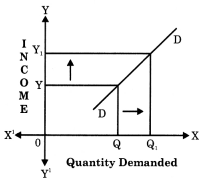Negative Income Elasticity:

1. When a change in income of the consumer brings about a change in demand of the commodity in the opposite direction then Income Elasticity is negative.
2. When income increases the demand decreases and when income decreases demand increases.
3. In case of inferior goods, the Income Elasticity is negative.
4. The slope of the Income Curve is negative. It slopes downward indicating that as income increases, demand decreases.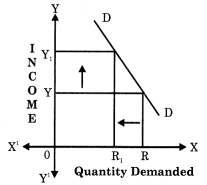Question 3.
Perfectly Inelastic Demand and Perfectly Elastic Demand.
Perfectly Inelastic Demand:

1. When a change in price brings no change in quantity demanded, then demand is said to be Perfectly Inelastic demand.
2. The numerical co-efficient is zero (e = 0).
3. The demand curve is vertical straight line parallel to Y-axis.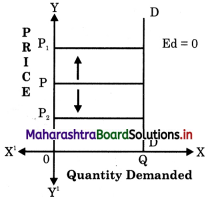Perfectly Elastic Demand:

1. When a small change in price brings infinite change in the quantity demanded, then demand is said to be Perfectly Elastic demand.
2. The numerical co-efficient is infinity (e = ∞).
3. The demand curve is a horizontal straight line parallel to X-axis.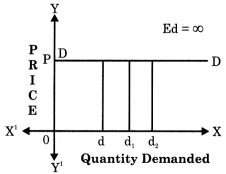Question 6.
Price Elasticity and Income Elasticity.
Price Elasticity:

1. Price Elasticity of demand refers to the degree of responsiveness of quantity demanded of a commodity to given change in price.
2. Symbolically: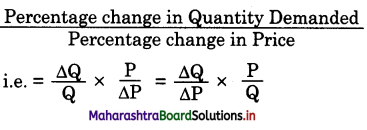3. There is inverse relation between price and demand.
4. Price Elasticity of demand are of five type :
1. Relatively elastic demand
2. Relatively inelastic demand
3. Unitary elastic demand
4. Perfectly elastic demand
5. erfectly inelastic demand

Income Elasticity:

1. Income elasticity of demand refers, to the degree of responsiveness of quantity demanded of the commodity to a given change in income.
2. Symbolically :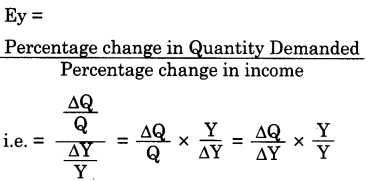3. There is direct relation between Income and demand.
4. Income elasticity of demand are of three types:
• Positive Income elasticity
• Negative Income elasticity
• Zero Income elasticity

Question 7.
Perfectly Inelastic Demand and Relatively Inelastic Demand.
Perfectly Inelastic Demand:

1. When a change in price brings no change in quantity demanded then, demand is said to be Perfectly Inelastic demand.
2. The numerical co-efficient is zero (e = 0).
3. The slope of the demand curve is straight line parallel to Y-axis.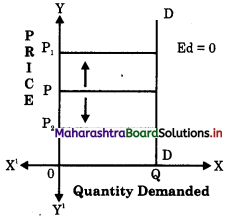4. Example : life saving drugs, salt, etc

Relatively Inelastic Demand:

1. When percentage change in quantity demanded is less than percentage change in price then, demand is said to be Relatively Inelastic demand.
2. The numerical co-efficient is less than one (e < 1).
3. The slope of the demand curve is steeper.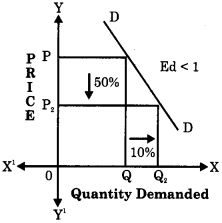4. Example: food grains

Question 8.
Perfectly Elastic Demand and Relatively Elastic Demand.
Perfectly Elastic Demand:

1. When with a small change in price brings infinite change in the quantity then it is said to be Perfectly Elastic demand.
2. The numerical co-efficient is infinity (e = ∞).
3. The demand curve is a horizontal straight line parallel to X-axis.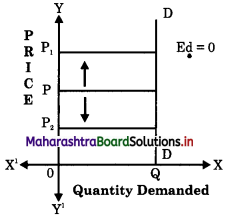Relatively Elastic Demand :

1. When percentage change in quantity demanded is greater than the percentage change in price demand then it is said to be Relatively Elastic demand.
2. The numerical co-efficient is greater than one (e > 1)
3. The demand curve slope is flatter.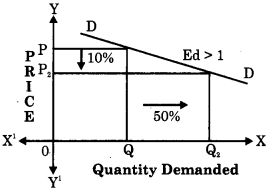Question 9.
Percentage Method and Total Outlay Method.
Percentage Method:

1. According to this method elasticity of demand is equal to the ratio of percentage change in the quantity demanded to percentage change in the price.
2. (1) If the result in one, the Elasticity is unitary.
(2) If the result is > 1 the demand is relatively elastic and
(3) If the result is < 1 then demand is relatively Inelastic.

Total Outlay Method:

1. Under this method Elasticity is measured by observing the changes in outlay in response to change in price.
2. Elasticity of demand is measured by the kind of changes in total expenditure.
• When with increase or decrease in price, the outlay remain constant the elasticity is equal to one.
• If outlay and price are inversely related then demand is relatively elastic.
• If outlay and price are directly related then demand is relatively Inelastic.

Question 1.
Explain the concept of Elasticity of Demand?
The concept of Price Elasticity was developed by great neo-classical economist Dr. Alfred Marshall in the year 1890.

According to Dr. Alfred Marshall, “The elasticity or responsiveness of demand in a market is great or small, according to the amount demanded which increases much or little for a given fall in price, and diminishes much or little for a given rise in price. ”
Elasticity of demand in fact refers to the degree of responsiveness of the quantity demanded of a commodity to change in the variable on which demand depends.

Question 2.
Which are the types of Elasticity of Demand.
Types of Elasticity of Demand :

1. Price Elasticity of Demand
2. Income Elasticity of Demand
3. Cross Elasticity of Demand

1. Price Elasticity of Demand : It is a ratio of proportionate change in the quantity demanded of a commodity to a given proportionate change in its price.
ed = $$\frac { Percentage change in Quantity Demanded }{ Percentage change in Price }$$
There are 5 types of Price Elasticity of demand i.e.

1. Perfectly Elastic demand (Ed = ∞)
2. Perfectly Inelastic demand (Ed = 0)
3. Unitary Elastic demand (Ed = 1)
4. Relatively Elastic demand (Ed > 1)
5. Relatively Inelastic demand (Ed < 1)

2. Income Elasticity of demand : It refers to the degree of responsiveness of a change in the quantity demanded to a change in the income only, other factors including price remaining unchanged.
$$\frac { Percentage change in Quantity Demanded }{ Percentage change in Income }$$
There are 3 types of Income elasticity i.e.:

1. Positive Income elasticity
2. Negative Income elasticity
3. Zero Income elasticity

3. Cross Elasticity of Demand :
It refers to a change in quantity demanded of one commodity due to a change in the price of other commodity i.e. complementary goods or substitute goods.
$$\frac { of commodity ‘X’ }{ Percentage change in Price of commodity ‘Y’ }$$

Cross elasticity of demand are of 3 types i.e.

1. Positive Cross elasticity
2. Negative Cross elasticity
3. Zero Cross elasticity

Question 3.
Explain any two types of price elasticity of demand.
Types of price elasticity of demand :
(a) Perfectly Elastic Demand (Ed = ∞) :

When a change in price leads to infinite change in quantity demanded of a commodity it is called as perfectly elastic demand. It is an extreme case, which is very rare. It is a theoretical concept not found in real life.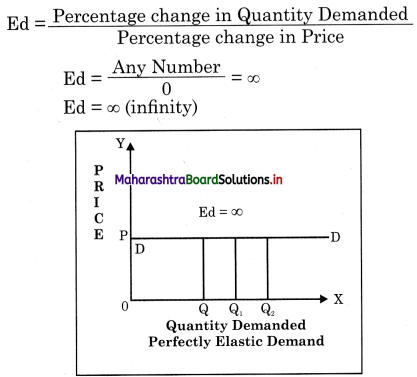In the diagram demand curve ‘DD’ is horizontal straight line parallel to X-axis,

(b) Perfectly Inelastic Demand (Ed = 0) : When a change in price has no effect on the quantity demanded of that commodity then it is called Perfectly Inelastic Demand. The demand does not respond to the change in price at all. For instance, if price changes by 10% and the demand does not change at all then e = 0.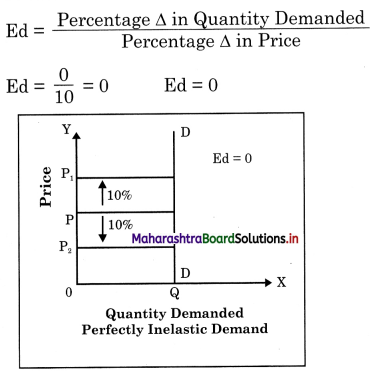In this case the numerical coefficient value is zero. It is also extreme case which rarely exists. Salt and life saving medicine are a ) few example of Perfectly Inelastic Demand as they are absolute necessity. The demand ) curve ‘DD’ is a vertical straight line parallel to Y-axis.

Question 4.
Explain the types of Income Elasticity.
(a) Positive Income Elasticity (Normal goods)
(b) Negative Income Elasticity (Inferior goods
(c) Zero Income Elasticity

(a) Positive Income Elasticity : In case of normal goods the income elasticity of demand is positive. When a change in income brings about a change in demand in same direction then income elasticity is positive. There is a direct relationship between income and quantity demanded. Normal goods which include necessities, comforts and luxuries have positive Income Elasticity.

(b) Negative Income Elasticity : Inferior goods have a negative income elasticity. As income increases the demand for inferior goods fall. There is an inverse relation between income and quantity demanded of inferior commodity. Inferior goods are substituted by superior goods.

(c) Zero Income Elasticity : When change in income does not have any effect on the demand for a commodity then the income elasticity is Zero – for E.g. goods like salt, pins etc.

Question 5.
Explain the types of Cross Elasticity of Demand.
(a) Positive Cross Elasticity (Substitutes)
(b) Negative Cross Elasticity (Complementary)
(c) Zero Cross Elasticity (Unrelated goods)

(a) Positive Cross Elasticity : Positive cross elasticity of demand takes place in the case of substitute such as tea and coffee, pepsi and coke. If the price of coffee rises it will lead to an increase in the demand for tea. Similarly a fall in price of coffee will cause a decrease in demand for tea. Thus price and demand move in the same direction in case of substitute.

(b) Negative Cross Elasticity : Negative cross elasticity takes place in the case of complementary goods. It takes place when a change in the price of (petrol) one complementary good brings about a change in demand for (car) another commodity in the opposite direction. For e.g. a rise in petrol prices may lead to fall in demand for car and vice-versa.

(c) Zero Cross Elasticity: Zero cross elasticity of demand takes place in case of unrelated goods. For instance a rise or fall in price of car may not affect the demand for books.

Question 6.
Explain the importance of the concept of Elasticity of Demand.
Or
Give the significance of Price elasticity of demand.
Importance of Concept of Elasticity of Demand:
1. To a Producer : It helps the producer to decide the price for his product. If the demand for his product is relatively inelastic, he will fix higher price and vice versa.

2. To Government : In order to raise additional revenue by means of new taxes like excise duties on commodities Govt, has to keep in mind the elasticity of demand for a commodity. So a high tax can be imposed on commodities like petrol or cigarettes, the demand for which is relatively inelastic.

3. In International Trade : If the foreign demand for exportable products of a country is relatively elastic, its export will rise much after a slight reduction in their prices. But if foreign demand is relatively inelastic, the exporters can raise their prices without much fall in exports.

4. Trade Union : Knowledge of elasticity of demand can help the trade union in wage bargaining. They can ask the employees { to pay higher wages to the workers by changing the price policy of their product. If the demand for the product produced by them is inelastic then the trade unionist may suggest the employer to raise the price, so as to earn higher profit. Which then can be used to pay higher wages.

5. Public Utility Services : It is the Government who takes the decision to distribute certain essential goods and services, which have inelastic demand. For eg. Indian Railways, bank service, water supply, etc. If such services are left to private sector they will exploit the consumers by charging high prices.

6. Devaluation : Devaluation measure is adopted by the government to promote exports and correct deficit in the balance of payment. Devaluation makes our goods cheaper in the foreign market. However, devaluation is successful only if exports have elastic demand.

7. Factor Pricing: It is helpful in determining the prices of various factors of production like rent for land, wages or salary for labour, interest for capital, etc.

4. State with reasons whether you agtae or disagree with the following statements;

Question 1.
Various factors influence elasticity of demand.
Yes, I agree with this statement.

• Nature of Commodity : By nature, commodities are classified as necessaries, comforts and luxuries. Normally demand for necessaries like food grains are relatively inelastic and for comforts and luxuries like diamond, perfumes, etc is relatively elastic.
• Availability of Substitutes : The larger the number of substitutes available for a commodity, the greater would be the elasticity. Demand for products like soap, soft drinks, detergents, tooth paste, etc. have many substitute so demand is elastic, ‘However, salt, garlic, onions have no substitute so demand is inelastic.
• Durability of the Commodity : The demand for durable goods like T.V., car, fridge, etc is relatively inelastic in the short run and elastic in the long run. Whereas the demand for perishable goods is relatively inelastic.
• Uses of Commodity : Single use commodities have less elastic demand and multi-use goods like coal, electricity, sugar, etc. have relatively elastic demand.
• Range of Price : The demand for commodities which are highly priced and will have a inelastic demand like AC, car, etc. Even very low priced goods have inelastic demand.
• Consumer’s Income : Generally if income is very high, the demand for over allcommodities tends to be relatively inelastic. The demand pattern of the rich people is rarely affected even when there is significant price change.

Question 2.
The price elasticity of demand depends only on the nature of commodity.

• Nature of Commodity : By nature, commodities are classified as necessaries, comforts and luxuries. Normally demand for necessaries like food grains are relatively inelastic and for comforts and luxuries like diamond, perfumes, etc is relatively elastic.
• Availability of Substitutes : The larger the number of substitutes available for a commodity, the greater would be the elasticity. Demand for products like soap, soft drinks, detergents, tooth paste, etc. have many substitute so demand is elastic, ‘However, salt, garlic, onions have no substitute so demand is inelastic.
• Durability of the Commodity : The demand for durable goods like T.V., car, fridge, etc is relatively inelastic in the short run and elastic in the long run. Whereas the demand for perishable goods is relatively inelastic.
• Uses of Commodity : Single use commodities have less elastic demand and multi-use goods like coal, electricity, sugar, etc. have relatively elastic demand.
• Range of Price : The demand for commodities which are highly priced and will have a inelastic demand like AC, car, etc. Even very low priced goods have inelastic demand.
• Consumer’s Income : Generally if income is very high, the demand for over allcommodities tends to be relatively inelastic. The demand pattern of the rich people is rarely affected even when there is significant price change.

Question 3.
The Income Elasticity of Demand is only positive.
No, I do not agree with this statement, s Income Elasticity of demand is not only positive but can also be negative or zero.

• Negative Income Elasticity : Inferior goods have a negative income elasticity. As income increases the demand for inferior goods fall. There is an inverse relation s between income and quantity demanded of inferior commodity. Inferior goods are substituted by superior goods.
• Zero Income Elasticity : When change in income does not have any effect on the demand for a commodity then the income elasticity is Zero – for E.g. goods like salt, pins etc.

Question 4.
Elasticity of Demand cannot be measured.
No, I do not agree with this statement,
Elasticity of demand can be measured in 3 methods i.e. Percentage method, Point method or Total Outlay method.

(i) Percentage Method : This method is also known as Arithmetic Method. In this method we compare the percentage of change in demand with percentage of change in price. When we divide the percentage of change in demand by the percentage of change in price, we get Elasticity of demand.

By applying the above formula we can calculate and find the type of elasticity. If the result is one, then elasticity is unitary, if it is more than one, then it is relatively elastic and if it is less than one, it is relatively inelastic.
Ed = $$\frac { Percentage change in Quantity demanded }{ Percentage change in Price }$$

(ii) Point Method : In this method we can find elasticity of demand at a point on a given demand curve by using the following formula –
Ed = $$\frac { Lower segment of the demand curve }{ Upper segment of the demand curve }$$

(iii) Total Outlay Method : According to this method, elasticity of demand is measured on the basis of expenditure incurred by consumer when the price of a commodity changes.
Total outlay can be calculated by multiplying the price with the quantity demanded i.e. Total Outlay = Price x Quantity Demanded

Question 1.
What is Elasticity of demand? Explain the types of Elasticity of Demand.

The concept of Price Elasticity was developed by great neo-classical economist Dr. Alfred Marshall in the year 1890.

According to Dr. Alfred Marshall, “The elasticity or responsiveness of demand in a market is great or small, according to the amount demanded which increases much or little for a given fall in price, and diminishes much or little for a given rise in price. ”
Elasticity of demand in fact refers to the degree of responsiveness of the quantity demanded of a commodity to change in the variable on which demand depends.

Types of Elasticity of Demand :

1. Price Elasticity of Demand
2. Income Elasticity of Demand
3. Cross Elasticity of Demand

1. Price Elasticity of Demand : It is a ratio of proportionate change in the quantity demanded of a commodity to a given proportionate change in its price.
ed = $$\frac { Percentage change in Quantity Demanded }{ Percentage change in Price }$$
There are 5 types of Price Elasticity of demand i.e.

1. Perfectly Elastic demand (Ed = ∞)
2. Perfectly Inelastic demand (Ed = 0)
3. Unitary Elastic demand (Ed = 1)
4. Relatively Elastic demand (Ed > 1)
5. Relatively Inelastic demand (Ed < 1)

2. Income Elasticity of demand : It refers to the degree of responsiveness of a change in the quantity demanded to a change in the income only, other factors including price remaining unchanged.
$$\frac { Percentage change in Quantity Demanded }{ Percentage change in Income }$$
There are 3 types of Income elasticity i.e.:

1. Positive Income elasticity
2. Negative Income elasticity
3. Zero Income elasticity

3. Cross Elasticity of Demand :
It refers to a change in quantity demanded of one commodity due to a change in the price of other commodity i.e. complementary goods or substitute goods.
Percentage change in Quantity demanded
$$\frac { of commodity ‘X’ }{ Percentage change in Price of commodity ‘Y’ }$$

Cross elasticity of demand are of 3 types i.e.

1. Positive Cross elasticity
2. Negative Cross elasticity
3. Zero Cross elasticity

Question 2.
What is Price Elasticity of Demand? Explain the types of Price Elasticity of Demand?
(A) Price Elasticity of Demand :
Price Elasticity of demand refers to the degree of responsiveness of quantity demanded of a commodity to the change in its price, other factors remaining constant. According to Marshall Price Elasticity of Demand (Ed) is the ratio of proportionate change in quantity demanded of a commodity to a \ given proportionate change in its price.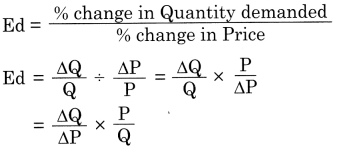∆ (delta) = Stands for change
∆Q = Change in Quantity demanded (New Qty. – Old Qty. demanded)
AP = Change in Price (New price – Old price)
P & Q = Original Price and Original Quantity Demanded

Using this formula price Elasticity of demand can be calculated for any set of data of demand and price. The value obtained may range from zero to infinity. Since quantity demanded has a negative relationship with price, the elasticity of demand so obtained will have negative sign, we ignore the negative sign altogether.

(B) Types of price elasticity of demand :
Types of price elasticity of demand :
(a) Perfectly Elastic Demand (Ed = ∞) :
When a change in price leads to infinite change in quantity demanded of a commodity it is called as perfectly elastic demand. It is an extreme case, which is very rare. It is a theoretical concept not found in real life.In the diagram demand curve ‘DD’ is horizontal straight line parallel to X-axis,

(b) Perfectly Inelastic Demand (Ed = 0) : When a change in price has no effect on the quantity demanded of that commodity then it is called Perfectly Inelastic Demand. The demand does not respond to the change in price at all. For instance, if price changes by 10% and the demand does not change at all then e = 0.In this case the numerical coefficient value is zero. It is also extreme case which rarely exists. Salt and life saving medicine are a ) few example of Perfectly Inelastic Demand as they are absolute necessity. The demand ) curve ‘DD’ is a vertical straight line parallel to Y-axis.

(c) Relatively Elastic Demand (Ed > 1):
When the proportionate or percentage change in the quantity demanded of a commodity is greater than proportionate or percentage change in its price then, demand is said to the relatively elastic.
Here the amount demanded of a commodity rises much after a slight fall in its price. For instance a 5% fall in price will bring a 10% rise in demand for that commodity.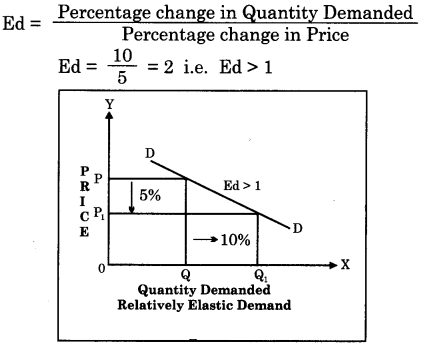The numerical coefficient value is greater than one and lies between 1 and ∞.

Example : Luxury goods BMW Car, air – conditioners, LED TV etc. will have relatively elastic demand. In the diagram the demand curve DD has a flatter slope. It slopes gradually.

(d) Relatively Inelastic Demand (Ed < 1):
When the proportionate or percentage change in quantity demanded of a commodity is less than proportionate or percentage change in its price then demand is said to be relatively inelastic. Here the degree of responsiveness of demand is very little to a given change in its price. For instance, if price falls by 10% then quantity demanded may rise by say 5%.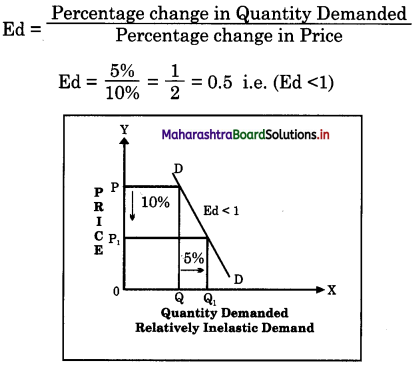The numerical coefficient value is less than one and lies between 0 and 1.
Example : The demand for necessary goods like wheat, rice, sugar etc. is said to be relatively inelastic as the demand is less responsive to a given change in price. In the diagram the demand curve DD has a rapid and steep slope.

(e) Unitary Elastic Demand (Ed = 1) :
When proportionate or percentage change in quantity demanded is exactly equal to proportionate or percentage change in price, then demand is said to be unitary elastic. For instance a 10% fall in price of a commodity leads to 10% rise in demand of that commodity.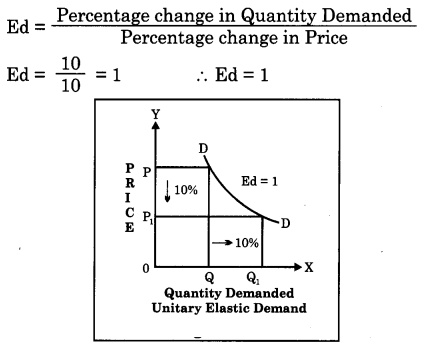In this case the demand curve slopes downwards uniformly. This demand curve is called a rectangular hyperbola as shown in the figure. The unity elasticity of demand is a dividing line to distinguish between relatively elastic demand and relatively inelastic demand.

Question 3.
Explain the different method of measuring Price Elasticity of Demand.
(A) Percentage Method or Ratio Method : This method is also known as Arithmetic Method. In this method} we compare the percentage of change in demand with percentage of change in price. When we divide the percentage of change in demand by the percentage of change in price, we get Elasticity of demand.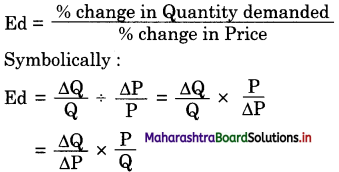ΔQ = Change in demand (Original – new ( quantity)
ΔP = Change in price (Original – new price)
Q = Original quantity
P = Original price
By applying the above formula we can ( calculate and find the type of elasticity. If the ) result is one, then elasticity is unitary. If it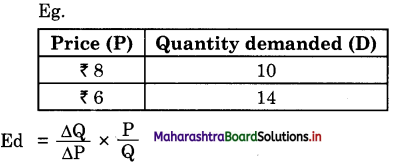[ ΔQ (Change in Qty) = (10 -14) = -4, Original Qty = 10, ΔP (Change in price) = (6 – 8) = -2, Original price = 8 ]
= $$\frac{-4}{-2} \times \frac{8}{10}=\frac{8}{5}$$
= 1.6 (e > 1)
So the commodity has Relatively Elastic Demand e > 1.
If the Answer was < 1 then it would have been relatively inelastic.

(B) Total Outlay Method : This method was introduced by Dr. Alfred Marshall. The limitation of this method is that in this method unlike ratio method, the exact numerical value of the elasticity of demand cannot be determined. According, to this method, the elasticity of demand is measured on the basis of expenditure incurred by consumer when the price of a commodity changes.

Total outlay or total expenditure can be calculated by multiplying the price with the quantity demanded (Price x Quantity demand = Total Expenditure). Depending upon the kind of change in total outlay, whether it increases, or decreases, or remain constant with the change in price we will be able to decide the type of elasticity. This can be explained with the following example:-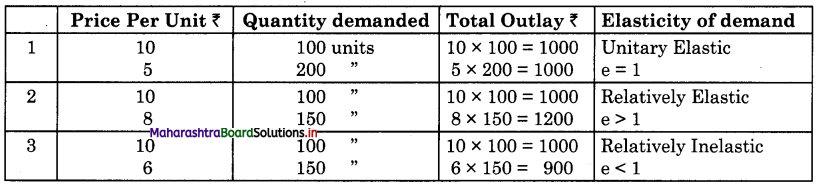(1) If the total outlay remains the same with a rise or fall in price then the demand is said to be unitary (e = 1) elastic.
(2) If the total outlay decreases with a rise in price and increases with a fall in price, the elasticity of demand is greater than one or Relatively Elastic e > 1.
(3) If the total outlay increases with a rise in price and decreases with a fall in price, then elasticity is less than one or relatively inelastic, e < 1.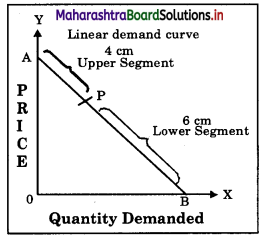(C) Point Method : In this method given by Prof. Marshall we will be able to find out elasticity at a point on a given demand curve.
To find out elasticity at any given point ‘P on a linear demand curve DD (Straight Line) extend the demand curve towards Y axis and X axis to meet at point A and B respectively as shown in the diagram. Then measure the distance of segment PA and segment PB. Then divide the distance of lower segment PB by upper segment PA.
Point Elasticity of demand = $$\frac { Lower segment of the demand curve }{ Upper segment of the demand curve }$$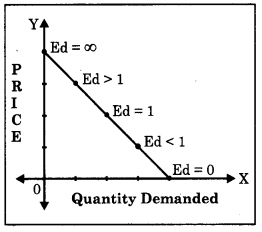Suppose the distance of lower segment PB is 6 cm and upper segment PA is 4 cm.
$$\frac{6}{4}=\frac{\mathrm{PB}}{\mathrm{PA}}$$ = 1.5
Then Elasticity at point “P” is 1.5 which is e > 1. So demand is Relatively Elastic. On a given linear demand curve elasticity of demand at different point is different as shown in the diagram.
Now to find elasticity of demand on a non-linear demand curve on a given point “P” as shown in the diagram. First draw a tangent line AB to meet Y and X axis at Point A and B. Then divide the
PB
lower Seg PB by the upper Seg PA i.e. $$\frac{\mathrm{PB}}{\mathrm{PA}}$$

Suppose distance PA’is 8 cm and PB is 4 cm, then ED = $$\frac{4}{8}$$ = 0.5 i.e. e < 1. It is a case of Relatively Inelastic demand.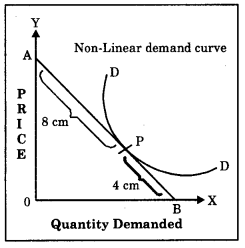Question 4.
Explain Ratio method and Geometric method of measuring price elasticity of demand.
(A) Percentage Method or Ratio Method : This method is also known as Arithmetic Method. In this method} we compare the percentage of change in demand with percentage of change in price. When we divide the percentage of change in demand by the percentage of change in price, we get Elasticity of demand.ΔQ = Change in demand (Original – new ( quantity)
ΔP = Change in price (Original – new price)
Q = Original quantity
P = Original price
By applying the above formula we can ( calculate and find the type of elasticity. If the ) result is one, then elasticity is unitary. If it[ ΔQ (Change in Qty) = (10 -14) = -4, Original Qty = 10, ΔP (Change in price) = (6 – 8) = -2, Original price = 8 ]
= $$\frac{-4}{-2} \times \frac{8}{10}=\frac{8}{5}$$
= 1.6 (e > 1)
So the commodity has Relatively Elastic Demand e > 1.
If the Answer was < 1 then it would have been relatively inelastic.

(C) Point Method : In this method given by Prof. Marshall we will be able to find out elasticity at a point on a given demand curve.
To find out elasticity at any given point ‘P on a linear demand curve DD (Straight Line) extend the demand curve towards Y axis and X axis to meet at point A and B respectively as shown in the diagram. Then measure the distance of segment PA and segment PB. Then divide the distance of lower segment PB by upper segment PA.
Point Elasticity of demand = $$\frac { Lower segment of the demand curve }{ Upper segment of the demand curve }$$Suppose the distance of lower segment PB is 6 cm and upper segment PA is 4 cm.
$$\frac{6}{4}=\frac{\mathrm{PB}}{\mathrm{PA}}$$ = 1.5
Then Elasticity at point “P” is 1.5 which is e > 1. So demand is Relatively Elastic. On a given linear demand curve elasticity of demand at different point is different as shown in the diagram.
Now to find elasticity of demand on a non-linear demand curve on a given point “P” as shown in the diagram. First draw a tangent line AB to meet Y and X axis at Point A and B. Then divide the
PB
lower Seg PB by the upper Seg PA i.e. $$\frac{\mathrm{PB}}{\mathrm{PA}}$$

Suppose distance PA’is 8 cm and PB is 4 cm, then ED = $$\frac{4}{8}$$ = 0.5 i.e. e < 1. It is a case of Relatively Inelastic demand.